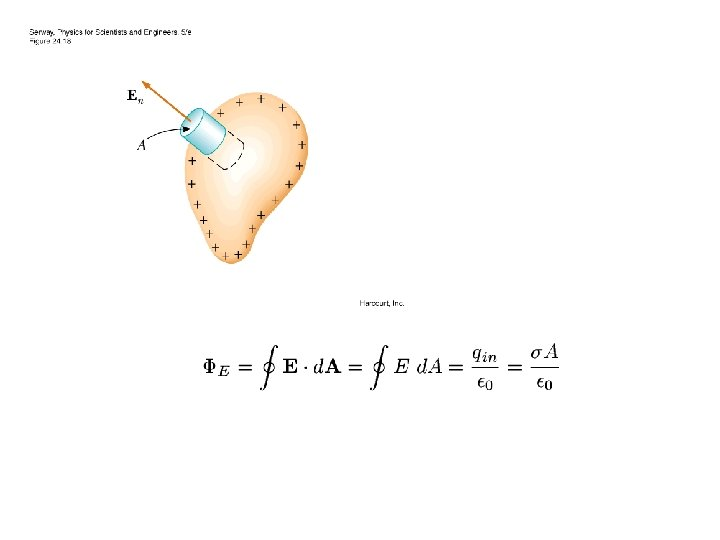# Electric Flux Proportional to the number of electric

• Slides: 10
Download presentation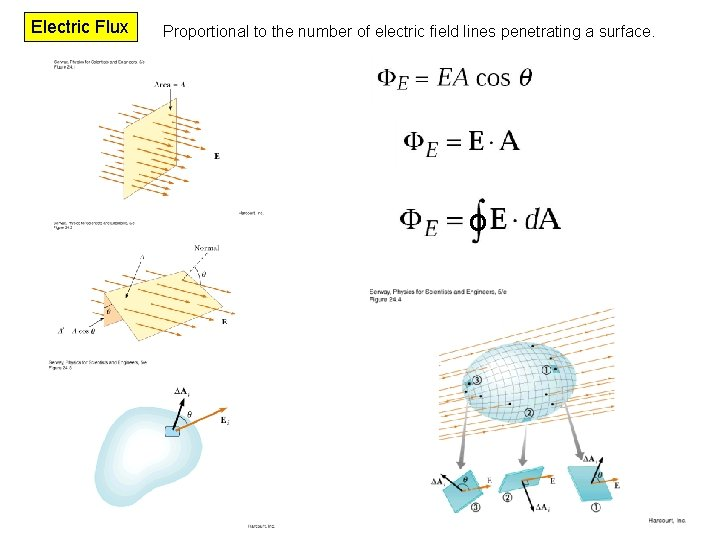Electric Flux Proportional to the number of electric field lines penetrating a surface. OGauss’s Law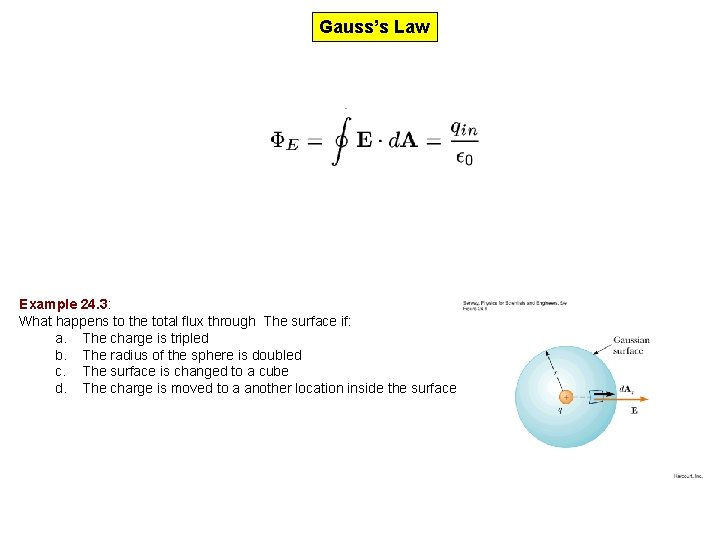Gauss’s Law Example 24. 3: What happens to the total flux through The surface if: a. The charge is tripled b. The radius of the sphere is doubled c. The surface is changed to a cube d. The charge is moved to a another location inside the surfaceApplication of Gauss’s Law Determine a surface such that: 1. Value of the electric field is constant over the surface 2. The dot product between E and d. A can be expressed as a simple algebraic product because E and d. A are parallel 3. The dot product is zero because E and d. A are perpendicular 4. The field is zero over the surface. Example 24. 4: Electric field due to a point chargeExample 24. 5: An insulating solid sphere of radius a carries a total charge Q. calculate: a. The magnitude of the electric filed at a point outside the sphere b. The magnitude of the electric filed at a point inside the sphereExample 24. 6: The electric field due to a thin spherical shell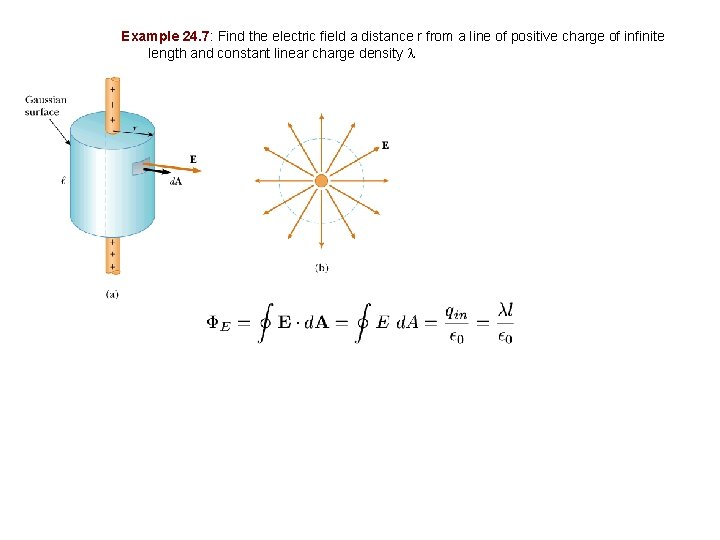Example 24. 7: Find the electric field a distance r from a line of positive charge of infinite length and constant linear charge density lExample 24. 8: Find the electric field due to a non-conducting , infinite plane of positive charge with uniform surface charge density s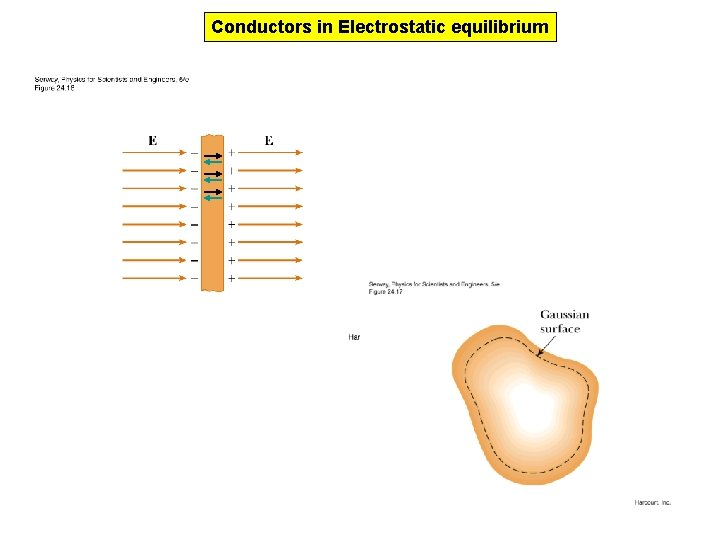Conductors in Electrostatic equilibrium